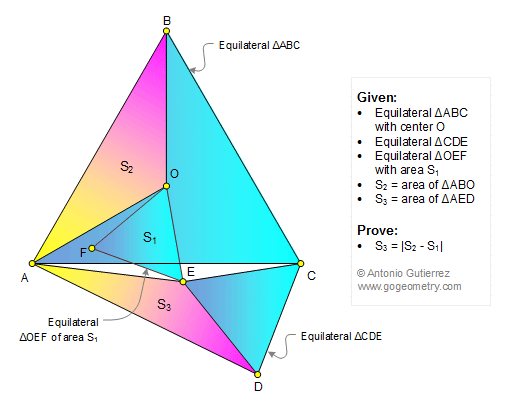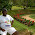## Wednesday, September 13, 2017

### Geometry Problem 1345: Three Equilateral Triangles, Center, Area, Common Vertex, 60 Degrees

Geometry Problem. Post your solution in the comment box below.
Level: Mathematics Education, High School, Honors Geometry, College.

Details: Click on the figure below.1.Trigonometry Solution

Let AB = a, OE = b and CE = c and
let < ACE = α.

S1 = √3b2/4 …(1) and
S2 = a2/(4√3)…(2)
being 1/3rd of S(ABC) = (√3a2/4)/3

S3 = S(ADC) – S(ACE) – S(CDE)
S3 = ½ ac.sin(α+60) - √3c2/4 – ½ ac.sinα
S3 = ½ ac.(1/2 sinα + √3/2 cosα – sinα) - √3c2/4
S3 = ½ ac(cos30.cosα – sin30.sinα) - √3c2/4
S3 = ½ ac cos(α+30) - √3c2/4…(3)

Now from ∆OCE, cos(α+30) = (a2/3 + c2 – b2)/(2ac/√3) …(4)

Substituting in (3),
S3 = √3/4. (a2/3 + c2 – b2) - √3c2/4
S3 = a2/(4√3) – √3b2/4
So S3 = S2 – S1 from (1) and (2).

If S1 > S2, then S3 = S1 – S2.

So generally S3 = IS2 – S1I

Sumith Peiris
Moratuwa
Sri Lanka

2.Do u have a pure geometry solution Antonio?